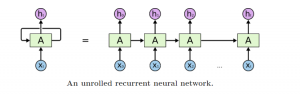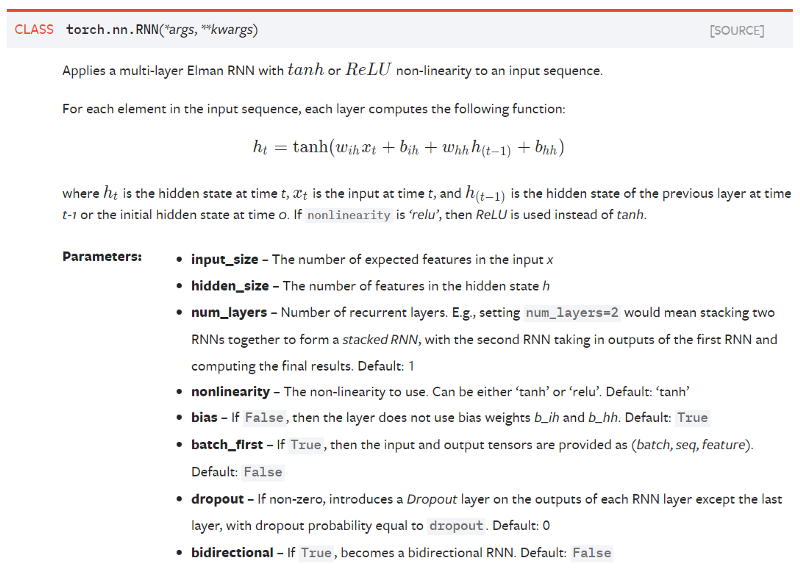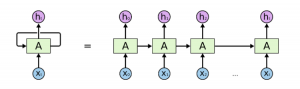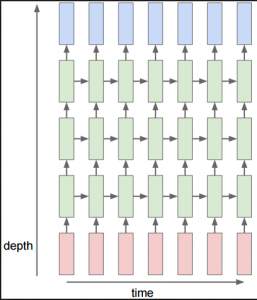Deep Learning

## Getting Started with NLP using the PyTorch Framework

March 19, 2019#### Natural Language Processing Using PyTorchIn this article we will be looking into the classes that PyTorch provides for helping with Natural Language Processing (NLP).

There are 6 classes in PyTorch that can be used for NLP related tasks using recurrent layers:

• torch.nn.RNN
• torch.nn.LSTM
• torch.nn.GRU
• torch.nn.RNNCell
• torch.nn.LSTMCell
• torch.nn.GRUCell

Understanding these classes, their parameters, their inputs and their outputs are key to getting started with building your own neural networks for Natural Language Processing (NLP) in Pytorch.

If you have started your NLP journey, chances are that you have encountered a similar type of diagram (if not, we recommend that you check out this excellent and often-cited article by Chris Olah — Understanding LSTM Networks):Such unrolled diagrams are used by teachers to provide students with a simple-to-grasp explanation of the recurrent structure of such neural networks. Going from these pretty, unrolled diagrams and intuitive explanations to the Pytorch API can prove to be challenging.Hence, in this article, we aim to bridge that gap by explaining the parameters, inputs and the outputs of the relevant classes in PyTorch in a clear and descriptive manner.

Pytorch basically has 2 levels of classes for building recurrent networks:

• Multi-layer classes — nn.RNN , nn.GRU andnn.LSTM
Objects of these classes are capable of representing deep bidirectional recurrent neural networks (or, as the class names suggest, one of more their evolved architectures — Gated Recurrent Unit (GRU) or Long Short Term Memory (LSTM) networks).
• Cell-level classes — nn.RNNCell , nn.GRUCell and nn.LSTMCell
Objects of these classes can represent only a single cell (again, a simple RNN or LSTM or GRU cell) that can handle one timestep of the input data. (Remember, these Cells don’t have cuDNN optimisation and thus don’t have any fused operations, etc.)

All the classes in the same level share the same API. Hence, understanding the parameters, inputs and outputs of any one of the classes in both the above levels is enough.

To make explanations simple, we will use the simplest classes — torch.nn.RNN and torch.nn.RNNCell

#### torch.nn.RNN :

We will use the following diagram to explain the API —#### Parameters:

• input_size — The number of expected features in the input x

This represents the dimensions of vector x[i] (i.e, any of the vectors from x to x[t] in the above diagram). Note that it is easy to confuse this with the sequence length, which is the total number of cells that we get after unrolling the RNN as above.

• hidden_size — The number of features in the hidden state h

This represents the dimension of vector h[i] (i.e, any of the vectors from h to h[t] in the above diagram). Together, hidden_size and input_size are necessary and sufficient in determining the shape of the weight matrices of the network.

• num_layers — Number of recurrent layers. E.g., setting num_layers=2would mean stacking two RNNs together to form a stacked RNN, with the second RNN taking in outputs of the first RNN and computing the final results. Default: 1

This parameter is used to build deep RNNs like these:Here red cells represent the inputs, green blocks represent the RNN cells and blue blocks represent the output

So for the above diagram, we would set the num_layers parameter to 3.

• nonlinearity — The non-linearity to use. Can be either ‘tanh’ or ‘relu’. Default: ‘tanh’

This is self-explanatory.

• bias — If False, then the layer does not use bias weights b_ih and b_hh. Default: True

In the Deep Learning community, some people find that removing/using bias does not affect the model’s performance. Hence, this boolean parameter.

• batch_first — If True, then the input and output tensors are provided as (batch, seq, feature). Default: False
• dropout — If non-zero, introduces a Dropout layer on the outputs of each RNN layer except the last layer, with dropout probability equal to dropout. Default: 0

This parameter is used to control the dropout regularisation method in the RNN architecture.

• bidirectional — If True, becomes a bidirectional RNN. Default: False

Creating a bidirectional RNN is as simple as setting this parameter to True!

So, to make an RNN in PyTorch, we need to pass 2 mandatory parameters to the class — input_size and hidden_size.

Once we have created an object, we can “call” the object with the relevant inputs and it returns outputs.

#### Inputs:

We need to pass 2 inputs to the object — input and h_0 :

• input — This is a tensor of shape (seq_len, batch, input_size). In order to work with variable lengthed inputs, we pack the shorter input sequences. See torch.nn.utils.rnn.pack_padded_sequence() or torch.nn.utils.rnn.pack_sequence() for details.
• h_0 — This is a tensor of shape (num_layers * num_directions, batch, hidden_size). num_directions is 2 for bidirectional RNNs and 1 otherwise. This tensor contains the initial hidden state for each element in the batch.

Outputs:

In a similar manner, the object returns 2 outputs to us — output and h_n :

• output — This is a tensor of shape (seq_len, batch, num_directions * hidden_size). It contains the output features (h_k) from the last layer of the RNN, for each k.
• h_n — This is a tensor of size (num_layers * num_directions, batch, hidden_size). It contains the hidden state for k = seq_len.

As mentioned before, both torch.nn.GRU and torch.nn.LSTM have the same API, i.e, they accept the same set of parameters and accept inputs in the same format and return out in the same format too.

#### torch.nn.RNNCell :

Since this represents only a single cell of the RNN, it accepts only 4 parameters, all of which have the same meaning as they did in torch.nn.RNN .

#### Parameters:

• input_size — The number of expected features in the input x
• hidden_size — The number of features in the hidden state h
• bias — If False, then the layer does not use bias weights b_ih and b_hh. Default: True
• nonlinearity — The non-linearity to use. Can be either ‘tanh’ or ‘relu’. Default: ‘tanh’

Again, since this is just a single cell of an RNN, the input and output dimensions are much simpler —

#### Inputs (input, hidden):

• input — this is a tensor of shape (batch, input_size) that contains the input features.
• hidden — this is a tensor of shape (batch, hidden_size) that contains the initial hidden states for each of the elements in the batch.

#### Output:

• h' — this is a tensor of shape (batch, hidden_size) and it gives us the hidden state for the next time step.

This was all about getting started with the PyTorch framework for Natural Language Processing (NLP). If you are looking for ideas on what is possible and what you can build, check out — Deep Learning for Natural Language Processing using RNNs and CNNs.### Free Resources

Browse our whitepapers, e-books, case studies, and reference architecture.

#### Have any questions?Deep Learning

## Getting Started with NLP using the PyTorch Framework

March 19, 2019 8 min read

#### Natural Language Processing Using PyTorchIn this article we will be looking into the classes that PyTorch provides for helping with Natural Language Processing (NLP).

There are 6 classes in PyTorch that can be used for NLP related tasks using recurrent layers:

• torch.nn.RNN
• torch.nn.LSTM
• torch.nn.GRU
• torch.nn.RNNCell
• torch.nn.LSTMCell
• torch.nn.GRUCell

Understanding these classes, their parameters, their inputs and their outputs are key to getting started with building your own neural networks for Natural Language Processing (NLP) in Pytorch.

If you have started your NLP journey, chances are that you have encountered a similar type of diagram (if not, we recommend that you check out this excellent and often-cited article by Chris Olah — Understanding LSTM Networks):Such unrolled diagrams are used by teachers to provide students with a simple-to-grasp explanation of the recurrent structure of such neural networks. Going from these pretty, unrolled diagrams and intuitive explanations to the Pytorch API can prove to be challenging.Hence, in this article, we aim to bridge that gap by explaining the parameters, inputs and the outputs of the relevant classes in PyTorch in a clear and descriptive manner.

Pytorch basically has 2 levels of classes for building recurrent networks:

• Multi-layer classes — nn.RNN , nn.GRU andnn.LSTM
Objects of these classes are capable of representing deep bidirectional recurrent neural networks (or, as the class names suggest, one of more their evolved architectures — Gated Recurrent Unit (GRU) or Long Short Term Memory (LSTM) networks).
• Cell-level classes — nn.RNNCell , nn.GRUCell and nn.LSTMCell
Objects of these classes can represent only a single cell (again, a simple RNN or LSTM or GRU cell) that can handle one timestep of the input data. (Remember, these Cells don’t have cuDNN optimisation and thus don’t have any fused operations, etc.)

All the classes in the same level share the same API. Hence, understanding the parameters, inputs and outputs of any one of the classes in both the above levels is enough.

To make explanations simple, we will use the simplest classes — torch.nn.RNN and torch.nn.RNNCell

#### torch.nn.RNN :

We will use the following diagram to explain the API —#### Parameters:

• input_size — The number of expected features in the input x

This represents the dimensions of vector x[i] (i.e, any of the vectors from x to x[t] in the above diagram). Note that it is easy to confuse this with the sequence length, which is the total number of cells that we get after unrolling the RNN as above.

• hidden_size — The number of features in the hidden state h

This represents the dimension of vector h[i] (i.e, any of the vectors from h to h[t] in the above diagram). Together, hidden_size and input_size are necessary and sufficient in determining the shape of the weight matrices of the network.

• num_layers — Number of recurrent layers. E.g., setting num_layers=2would mean stacking two RNNs together to form a stacked RNN, with the second RNN taking in outputs of the first RNN and computing the final results. Default: 1

This parameter is used to build deep RNNs like these:Here red cells represent the inputs, green blocks represent the RNN cells and blue blocks represent the output

So for the above diagram, we would set the num_layers parameter to 3.

• nonlinearity — The non-linearity to use. Can be either ‘tanh’ or ‘relu’. Default: ‘tanh’

This is self-explanatory.

• bias — If False, then the layer does not use bias weights b_ih and b_hh. Default: True

In the Deep Learning community, some people find that removing/using bias does not affect the model’s performance. Hence, this boolean parameter.

• batch_first — If True, then the input and output tensors are provided as (batch, seq, feature). Default: False
• dropout — If non-zero, introduces a Dropout layer on the outputs of each RNN layer except the last layer, with dropout probability equal to dropout. Default: 0

This parameter is used to control the dropout regularisation method in the RNN architecture.

• bidirectional — If True, becomes a bidirectional RNN. Default: False

Creating a bidirectional RNN is as simple as setting this parameter to True!

So, to make an RNN in PyTorch, we need to pass 2 mandatory parameters to the class — input_size and hidden_size.

Once we have created an object, we can “call” the object with the relevant inputs and it returns outputs.

#### Inputs:

We need to pass 2 inputs to the object — input and h_0 :

• input — This is a tensor of shape (seq_len, batch, input_size). In order to work with variable lengthed inputs, we pack the shorter input sequences. See torch.nn.utils.rnn.pack_padded_sequence() or torch.nn.utils.rnn.pack_sequence() for details.
• h_0 — This is a tensor of shape (num_layers * num_directions, batch, hidden_size). num_directions is 2 for bidirectional RNNs and 1 otherwise. This tensor contains the initial hidden state for each element in the batch.

Outputs:

In a similar manner, the object returns 2 outputs to us — output and h_n :

• output — This is a tensor of shape (seq_len, batch, num_directions * hidden_size). It contains the output features (h_k) from the last layer of the RNN, for each k.
• h_n — This is a tensor of size (num_layers * num_directions, batch, hidden_size). It contains the hidden state for k = seq_len.

As mentioned before, both torch.nn.GRU and torch.nn.LSTM have the same API, i.e, they accept the same set of parameters and accept inputs in the same format and return out in the same format too.

#### torch.nn.RNNCell :

Since this represents only a single cell of the RNN, it accepts only 4 parameters, all of which have the same meaning as they did in torch.nn.RNN .

#### Parameters:

• input_size — The number of expected features in the input x
• hidden_size — The number of features in the hidden state h
• bias — If False, then the layer does not use bias weights b_ih and b_hh. Default: True
• nonlinearity — The non-linearity to use. Can be either ‘tanh’ or ‘relu’. Default: ‘tanh’

Again, since this is just a single cell of an RNN, the input and output dimensions are much simpler —

#### Inputs (input, hidden):

• input — this is a tensor of shape (batch, input_size) that contains the input features.
• hidden — this is a tensor of shape (batch, hidden_size) that contains the initial hidden states for each of the elements in the batch.

#### Output:

• h' — this is a tensor of shape (batch, hidden_size) and it gives us the hidden state for the next time step.

This was all about getting started with the PyTorch framework for Natural Language Processing (NLP). If you are looking for ideas on what is possible and what you can build, check out — Deep Learning for Natural Language Processing using RNNs and CNNs.### Free Resources

Browse our whitepapers, e-books, case studies, and reference architecture.